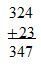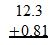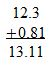# Adding and Subtracting Decimals: Examples & Word Problems

An error occurred trying to load this video.

Try refreshing the page, or contact customer support.

Coming up next: Multiplying and Dividing Decimals: Examples & Word Problems

### You're on a roll. Keep up the good work!

Replay
Your next lesson will play in 10 seconds
• 2:45 Example
• 3:36 Subtracting Decimals
• 4:58 Subtraction Example
• 6:02 Lesson Summary
Save Save

Want to watch this again later?

Timeline
Autoplay
Autoplay
Speed Speed Audio mode

#### Recommended Lessons and Courses for You

Lesson Transcript
Instructor: Yuanxin (Amy) Yang Alcocer

Amy has a master's degree in secondary education and has taught math at a public charter high school.

In math, we sometimes have to deal with decimal numbers instead of our nice whole numbers. Watch this video lesson to learn the one thing that you have to take into consideration when adding and subtracting decimals.

Adding and subtracting decimal numbers, numbers with a decimal point, isn't all that different from adding and subtracting whole numbers. Think about the steps you take to add and subtract whole numbers. Say you want to add 324 to 23. What do you do?

If you were doing it by hand, you would rewrite the problem so that your numbers are stacked on top of each other. You write the numbers so that the last digit is lined up with each other. For 324 + 23, you write it so that the 4 is on top of the 3. Then the other numbers follow suit.

You also have a line underneath the 23 and a + sign to the left of the 23. You go ahead and add the numbers digit-by-digit, starting from the right and going to the left. If any two digits add up to 10 or more, you carry the digit that is in the tens spot. So first we add 4 + 3 to get 7. We write the 7 directly underneath the 4 and the 3 under the line. And we continue until we reach the last digit. Since there is no number underneath the 3 in 324, we can write a 0 as a placeholder and to let us know that the value is 0. Our answer here is 347.To add decimals, we follow the same process except now when we line up our numbers, we use the decimal point as our point of reference instead of our last digit. For example, to add 12.3 to 0.81, we write the numbers stacked on top of each other, lining up the numbers according to the decimal point.Just like we can write 0s as placeholders when we add whole numbers, we can do the same thing when adding decimals. We notice that there is no number above the 1, so we can write a 0 there as a placeholder and to let us know that the value of this spot is 0. We then can proceed with the addition like we usually do. When we get to the decimal point, we also write the decimal point in our answer directly underneath.

We begin with 0 + 1 to get 1. Then we have 3 + 8, which is 11. Because this number is 10 or more, we carry the digit to the tens spot, the 1, and write the digit in the ones spot in the answer area. We write 1 down and carry the 1 over to the 2 + 0 so that we are adding an additional 1 to this spot.

So 2 + 0 becomes 2 + 1 and we get 3. Because we passed the decimal point, we make sure we write this decimal point in our answer between the 3 and the 1. Next, we have just a single 1. There is no number underneath, so it is a 0. 1 + 0 is 1. Our answer is 13.11.## Example

Let's try a problem. Our problem is telling us that Sarah is at the store and wants to purchase two candies. The large candy costs \$3.14 and the small candy costs \$0.34. How much is the total?

To answer this problem, we need to add 3.14 to 0.34. To do that, we rewrite the problem so that the numbers are stacked on top of each other with the decimal point lined up. We can write in any 0s that we need for placeholders if needed. Then we go ahead and add the digits starting from the right and working our way to the left. We have 4 + 4 = 8. 1 + 3 = 4. Decimal point. 3 + 0 = 3. Our answer is 3.48.

## Subtracting Decimals

Subtracting decimals, just like adding decimals, is similar to subtracting whole numbers. Just like adding decimals, the only difference is that we line up according to the decimal point instead of the last digit. Just like when we subtract whole numbers, we also write the numbers stacked on top of each other with the number we are subtracting on the bottom.

To unlock this lesson you must be a Study.com Member.

### Register to view this lesson

Are you a student or a teacher?

#### See for yourself why 30 million people use Study.com

##### Become a Study.com member and start learning now.
Back
What teachers are saying about Study.com

### Earning College Credit

Did you know… We have over 200 college courses that prepare you to earn credit by exam that is accepted by over 1,500 colleges and universities. You can test out of the first two years of college and save thousands off your degree. Anyone can earn credit-by-exam regardless of age or education level.